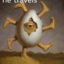MathematicsOpenStudy (anonymous):

evaluate the integral by making the given substitution. int[(5*sin*sqrt(x))/sqrt(x)] , u=sqrt(x)OpenStudy (anonymous):

forgot the dx! int[(5*sin*sqrt(x))/sqrt(x)] *dx , u=sqrt(x)OpenStudy (anonymous):

$5\int \frac{\sin(\sqrt{x})}{\sqrt{x}}dx$OpenStudy (anonymous):

$u=\sqrt{x}$ $du=\frac{1}{2\sqrt{x}}dx$ $2du=\frac{dx}{\sqrt{x}}$ $10\int \sin(u)du$OpenStudy (anonymous):

$-10\cos(u)$ $-10\cos(\sqrt{x})+C$OpenStudy (anonymous):

check is to take the derivative and see that you get the integrandOpenStudy (anonymous):

thank you!!OpenStudy (anonymous):

yw

Latest QuestionsShelbiLW: A 3.0 m uniform beam of mass 15 kg is pivoted 1.0 m from the end as shown below. A 35 kg child sits 0.
5 hours ago 0 Replies 0 Medalsryleighmullins22: Answer the question below using the following amortization table. Month Principal Paid Interest Paid Balance 21 \$654.
5 hours ago 0 Replies 0 MedalsTonycoolkid21: help
5 hours ago 8 Replies 0 MedalsTonycoolkid21: help
5 hours ago 15 Replies 3 MedalsRx1nyDxyz: Help If the supply of a product increases, then the price decreases*** the price
7 hours ago 21 Replies 1 Medalshototodorokii: (not from a test/quiz)Nomenclature is governed by certain universal rules. Which
7 hours ago 0 Replies 0 Medalscrispyrat: A guide to sqrt and cbrt
6 hours ago 21 Replies 3 MedalsxXQuintonXx: help please(ss below)
7 hours ago 5 Replies 1 Medalajfknkjfa: Prove that the two circles shown below are similar. (10 points) Circle C is shown with a center at negative 3, 1 and a radius of 4.
7 hours ago 3 Replies 1 Medalrxcklesskaisher: idk wdh dis is
8 hours ago 2 Replies 0 Medals